# Operators in Python

Challenge Inside! : Find out where you stand! Try quiz, solve problems & win rewards!
Learn via video coursePython Course for Beginners With Certification: Mastering the Essentials
By Rahul JanghuFree
4.90Enrolled: 1000Python Course for Beginners With Certification: Mastering the Essentials
Rahul JanghuFree
4.90
Enrolled: 1000

## Overview

Operators in Python are special symbols that carry arithmetic or logical operations. The value that the operator operates on is called the operand. In Python, there are seven different types of operators: arithmetic operators, assignment operators, comparison operators, logical operators, identity operators, membership operators, and boolean operators.

## Introduction to Operators in Python

Have you learned about addition and subtraction in school? Of course, right! These are known as operations. When you perform some calculations on some data and get a new value, we say you performed operations. These can be addition, subtraction, multiplication, division, comparing two values, and the list. Can you guess by the name; what are operators?

The symbol you use in addition, +, is an operator. Similarly, for other operations, we’ve several other operators to use.

If you cannot solve any equations or sum of Mathematics, Python can be your best friend. Python can solve any sum in less than a second. Write one line of code in Python, and you get your answer. Let’s understand how to get our answer quickly.

The first thing you need to know is about Operands. Operands are the quantities on which you want to operate. The image below represents an addition operation on two operands: '3' and '2', and you get '5' after the operation.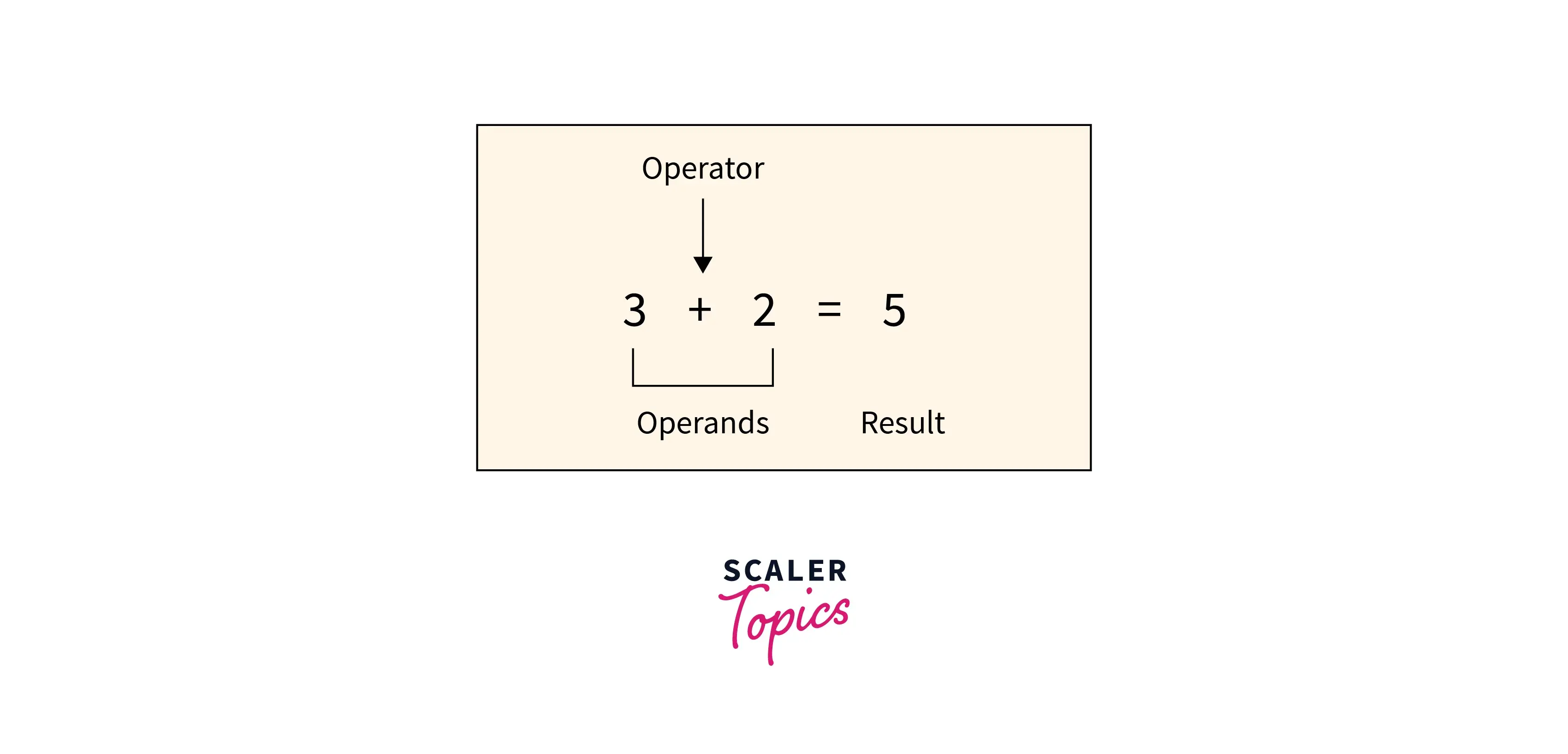## Types of Operators in Python

Operators are divided into 7 categories in Python according to their type of operation.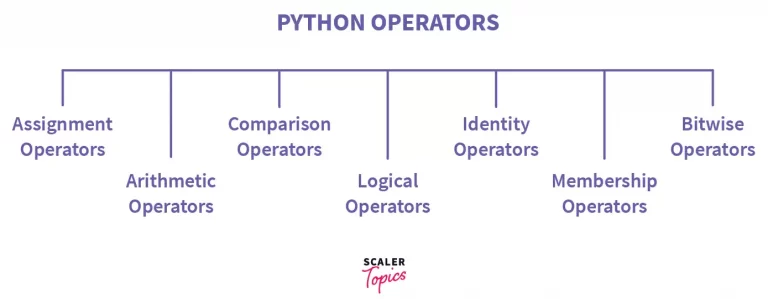Let’s see each one of them in detail:

### 1. ARITHMETIC OPERATORs in Python

Arithmetic operators are what we have used in our school life. There are 7 types possible under arithmetic operations: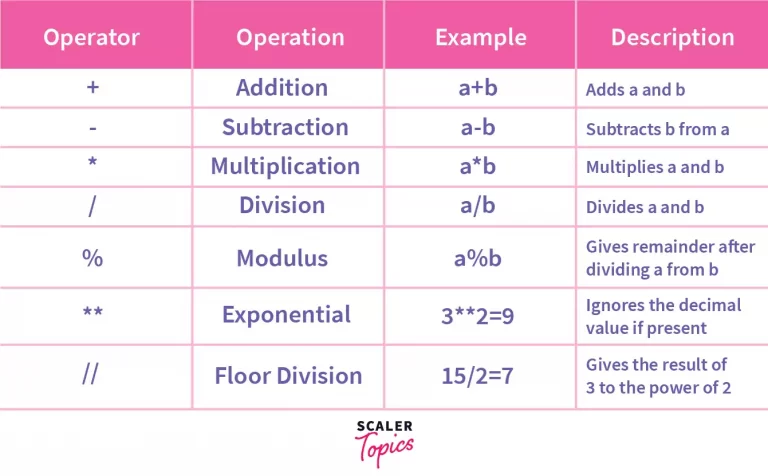### 2. ASSIGNMENT OPERATORs in Python

These are operators used for assigning values to a variable. The values to be assigned must be on the right side, and the variable must be on the left-hand side of the operator. There are various ways of doing it. Let’s see each one of them in detail: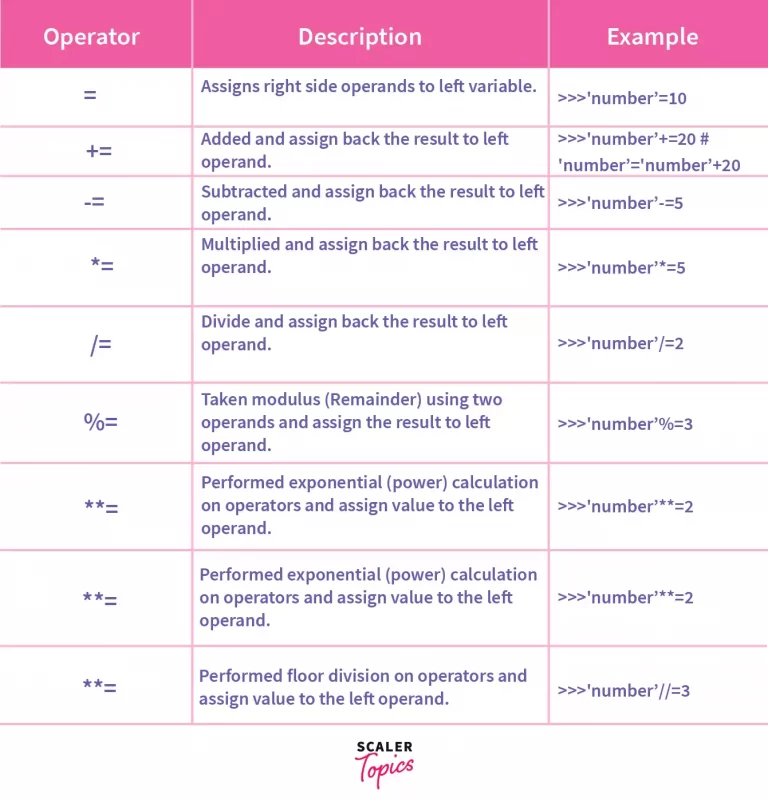### 3. COMPARISON OPERATORs in Python

These operators compare the value of the left operand and the right operand and return either True or False. Let’s consider a equals 10 and b equals 20.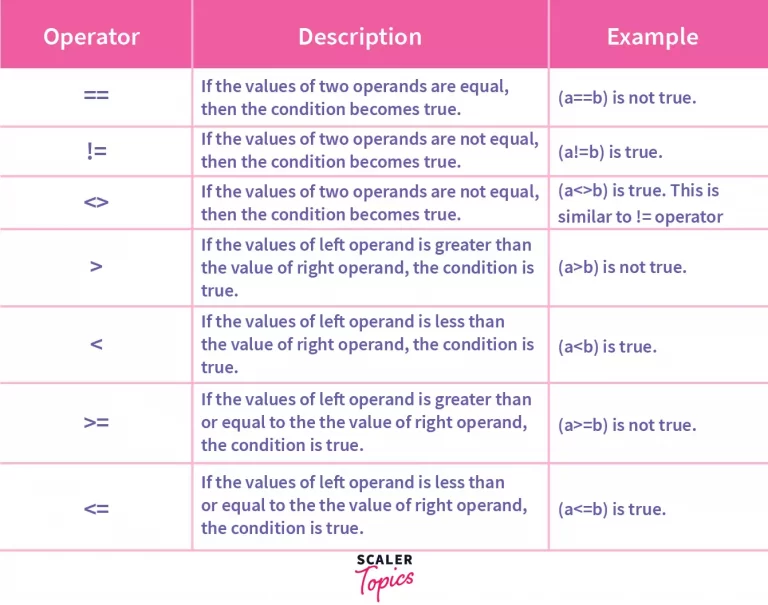### 4. LOGICAL OPERATORs in Python

Let’s understand the logical operator with an example. You decide you go to school only if your friend also goes. So either you both go to school or you both don’t. And that’s how an and statement works. If one of the conditions gives False as output, no matter what the other conditions are, the entire statement will return false.

Now in the or statement, if any one of the statements is True, the entire statement returns True. For example, you go to school if your friend comes or you’ve got a bike. So either of the statements can be True.

The not operator is used to reverse the result, i.e., to make False as True and vice versa.

These operators in Python are used when different conditions are to be met together. There are various types in it: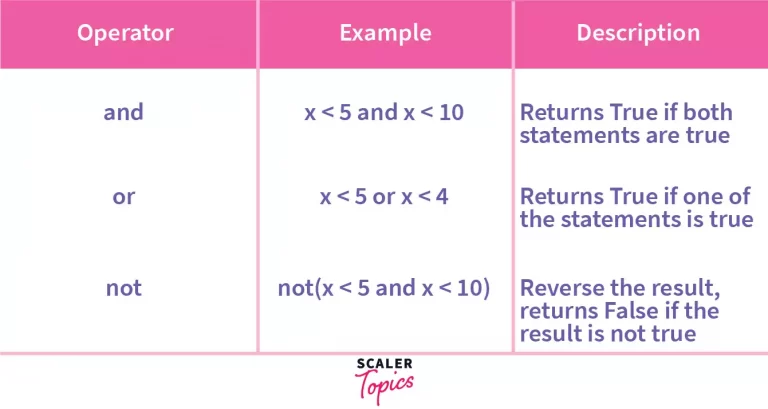Let’s suppose the value of x is 3, and we do the operation of and on it. It will return True because 3 is less than 5 and 10. For the or operation, it will return True if at least one of the conditions is True. The not operation is an operator which negates the value. For example, if the answer is True, negation is False.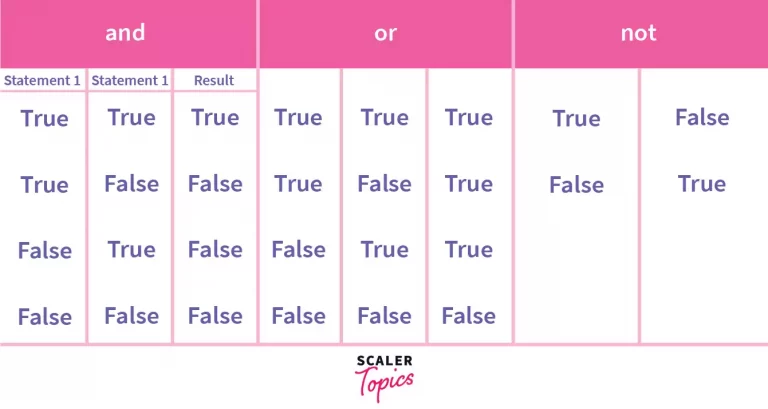### 5. IDENTITY OPERATORs in Python

These operators compare the left and right operands and check if they are equal, the same object, and have the same memory location.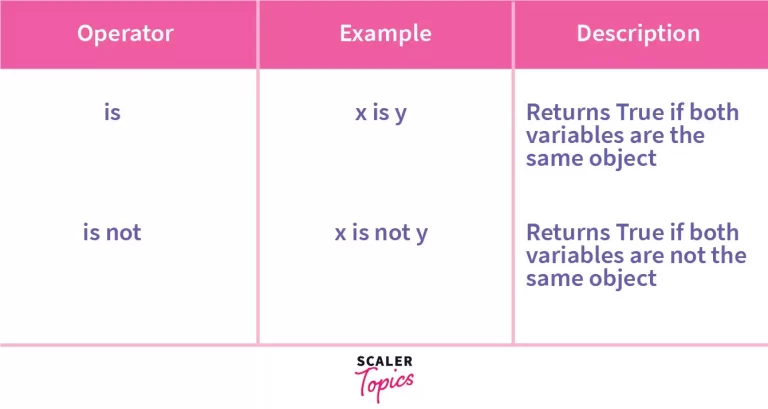#### Example

Code:

Output:

The first print statement checks if number1 and number2 are equal or not; if they are, it gives output as True; otherwise, False. The next print statement checks for number1 and number3. To return True, number1 and number3 shouldn’t be equal.

### 6. MEMBERSHIP OPERATORs in Python

These operators search for the value in a specified sequence and return True or False accordingly. If the value is found in the given sequence, it gives the output as True; otherwise False. The not in operator returns true if the value specified is not found in the given sequence. Let’s see an example:In the first example, 5 is present in the given sequence. Hence it will return True. And in the next example, 5 is not present in the sequence; hence, the not in operator will return True.

### 7. BITWISE OPERATORs in Python

These operators perform operations on binary numbers. So if the number given is not in binary, the number is converted to binary internally, and then an operation is performed. These operations are generally performed bit by bit.

Let’s consider a simple example considering a = 4 and b = 3. At first, both the values are considered in binary format, so 4 in binary is 0100, and 3 in binary is 0011. The bit-by-bit operation is performed when the & operation is performed.

So the last digits of both the numbers are compared, and it returns 1 only if both the numbers are 1; otherwise, 0. Likewise, all the bits are compared one by one and then provide the answer.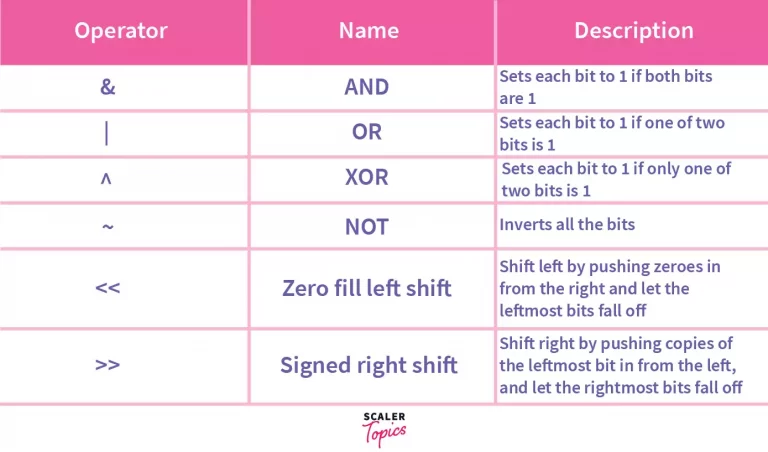#### Let’s see an example –

Code:

Output:

• For the and operation, the results become 0 because no same bit in 4 or 3 is 1.
• In the case of the or operator, if at least one bit is 1, then it returns 1. So here, in the above example, it returns 0111, which is 7.
• If both the bits are the same in the xor operation, it returns 0, otherwise 1. So, here it returns, 0111, that is 7.
• The ~ works on only one operator. It always returns -(n+1), hence -4.
• The << operator shifts the digits but one bit to the left, giving the output as 1000, which is 8. Similarly, the >> operator shifts the bits by one bit to the right side, giving 0010, which is 2, as output.

## Conclusion

• Operators are the backbone of Python.
• Operators are widely used for adding two numbers to assign value to a variable.
• The different types of operators are arithmetic operators, assignment operators, comparison operators, logical operators, identity operators, membership operators, and boolean operators.

Now that we know what operators are, we are one step further in writing better and more efficient codes. So, start implementing all the operators in your codes that you have learned today.